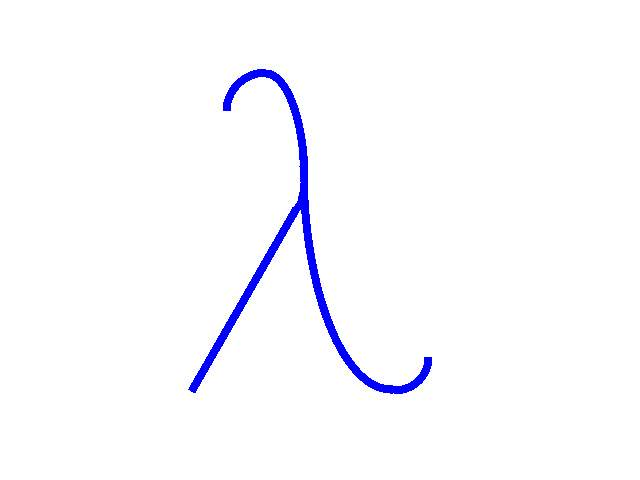# MiniLispMany years working with Lush have taken their toll. There are many things I really dislike doing in C or C++ when I know how easy they would be in Lisp. The essential lisp data structure is called the S-Expression. S-Expressions can represent lots of complicated things using a simple printable format. These are the main reason why many people find XML or JSON appealing. But I keep returning to S-Expression because they are so much simpler.

## MiniExp

At some point I decided to simply write a C++ library to handle the basic lisp data structure: S-expressions. The implementation fits in a single C++ file, including a garbage collector and a pretty printer. The full documentation is available as comments in the header file miniexp.h

MiniExp handles four basic types of S-expressions:

• Integers, in range [-229…229-1].
• Symbols, which are small strings represented by a unique handle.
• Pairs, which are the basic components of lists.
• Objects, which encapsulate any C++ object derived from class miniobj_t

Examples:

minivar_t a_symbol = miniexp_symbol("foo");
minivar_t a_number = miniexp_number(23);
minivar_t a_list = miniexp_cons(a_symbol, miniexp_cons(a_number, miniexp_nil));
minivar_t an_object = miniexp_string("Strings are implemented as objects.");

The type of the S-expression is miniexp_t. But we often store them into variables of type minivar_t. The difference is garbage collection. The garbage collector preserves all S-expressions stored in a minivar_t and all S-expressions recursively pointed by preserved S-expresssions.

To print a S-expression, one could write something like:

if (miniexp_numberp(exp))
printf("%d", miniexp_to_int(exp));
else if (miniexp_symbolp(exp))
printf("%s", miniexp_to_name(exp));
else if (miniexp_consp(exp))
....

But there is an easier way:

miniexp_print(exp);

and a prettier way:

miniexp_pprint(exp, 72);  // 72 is the target number of columns

You can also read S-expressions from the standard input

minivar_t exp = miniexp_read();

See the documentation in file miniexp.h for more details.

## MiniLisp

During the development of miniexp.h and miniexp.cpp, it appeared that the best way to test s-expressions was to make the last step and program a complete lisp interpreter.

Let's see what it can do:

MiniLisp, (C) 2005, Leon Bottou.
Available under the GNU General Public Licence.
(+ 2 3)
= 5
(defun (square x) (* x x))
= square
(square (+ 2 3))
= 25
(defun (sum l) (if (consp l) (+ (car l) (sum (cdr l))) 0))
= sum
(sum '(1 8 7 3))
= 19
(pretty sum)
(defun (sum l) (if (consp l) (+ (car l) (sum (cdr l))) 0))
= ()
(pretty pretty)
(defmacro (pretty f)
(let ((s (if (symbolp f) (list (list quote f)))))
(list (quote let)
(list (list (quote s) (list (quote funcdef) f . s)))
(list (quote pprint) (quote s) 72)
() ) ) )
= ()

The interpreter is very slow because symbol lookup is not optimized. But it makes a nice example of using MiniExp.

The documentation is in file miniexp.h. You can also check the MiniLisp source: